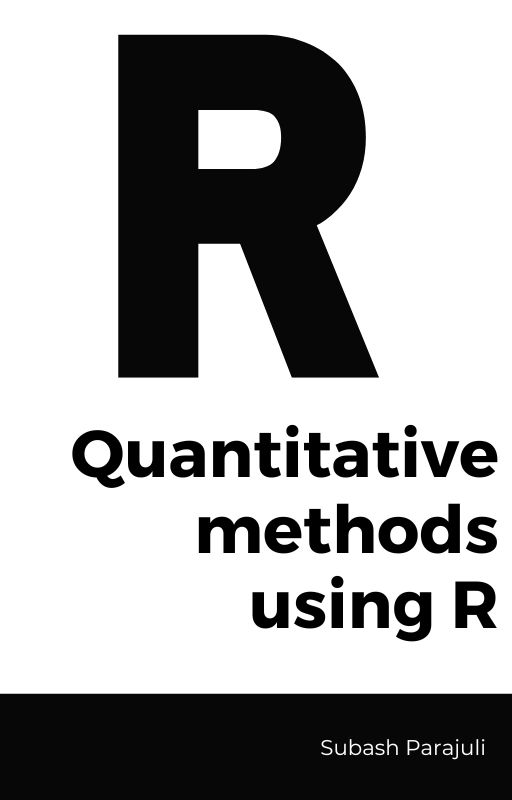# Welcome!This book covers practical worked out examples which you can easily apply to your data set and also includes a discussion on how the example is working. We will cover descriptive and basic inferential statistics, including graphs, frequency distributions, central tendency, dispersion, probability, hypothesis testing, tests of mean differences, correlation, simple regression, and chi-square tests. This book is designed to facilitate graduate students of Educational Psychology to develop their knowledge and understanding of various statistical concepts and procedures in R programming as a supplement resource.

## General Objectives

This book is based on a 3 credit semester course “Quantitative Methods - I” as taught in University of Oklahoma in Fall 2022. After thoroughly following the procedures and going through the examples , a learner will be able to

• Identify variables correctly falling at different scales of measurement.

• Identify appropriate techniques for analyzing data when presented with variables with different measurement characteristics.

• Set up and manage data sets containing variables in R and RStudio.

• Analyze data sets using quantitative techniques using R.

• Distinguish between null and alternative (research) hypotheses.

• Distinguish between a directional and non-directional hypothesis.

• Demonstrate the concepts of “statistical significance” and “effect size”.

• Analyze the effects of sampling (e.g., size, strategies) on inferences concerning population estimates.

• Interpret the results of statistical analyses.